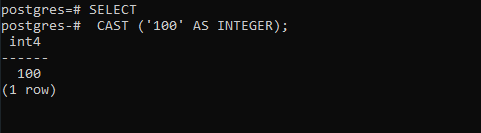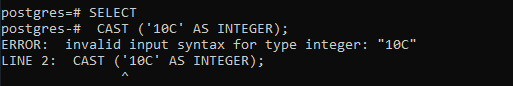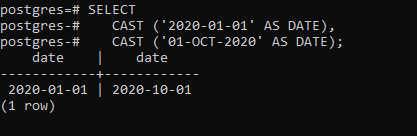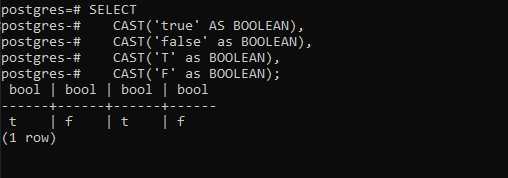# PostgreSQL – CAST

• Last Updated : 28 Aug, 2020

PostgreSQL supports a CAST operator that is used to convert a value of one type to another.

```Syntax: CAST ( expression AS target_type );

```

Let’s analyze the above syntax:

• First, specify an expression that can be a constant, a table column, an expression that evaluates to a value.
• Then, specify the target data type to which you want to convert the result of the expression.

Example 1:

The following statement converts a string constant to an integer:

```SELECT
CAST ('100' AS INTEGER);

```

Output:If the expression cannot be converted to the target type, PostgreSQL will raise an error. See the following:

```SELECT
CAST ('10C' AS INTEGER);

```

This will result to the below-depicted error:Example 2:

This example uses the CAST to convert a string to a date:

```SELECT
CAST ('2020-01-01' AS DATE),
CAST ('01-OCT-2020' AS DATE);

```

Output:Example 3:

This example uses the CAST() to convert the string ‘true’, ‘T’ to true and ‘false’, ‘F’ to false:

```SELECT
CAST('true' AS BOOLEAN),
CAST('false' as BOOLEAN),
CAST('T' as BOOLEAN),
CAST('F' as BOOLEAN);

```

Output:My Personal Notes arrow_drop_up